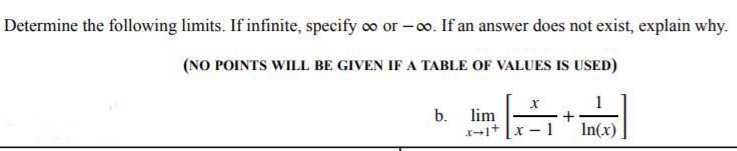### ¿Todavía tienes preguntas de matemáticas?

Pregunte a nuestros tutores expertos
Algebra
PreguntaDetermine the following limits. If infinite, specify $$\infty$$ or $$- \infty$$ . If an answer does not exist, explain why. (NO POINTS WILL BE GIVEN IF A TABLE OF VALUES IS USED) b. $$\lim _ { x \rightarrow 1 ^ { + } } [ \frac { x } { x - 1 } + \frac { 1 } { \ln ( x ) } ]$$

$$\infty$$